# 【C语言】程序环境和预编译# 一、程序的翻译环境和运行环境

## 1.翻译环境（编译（预编译、编译、汇编）+链接）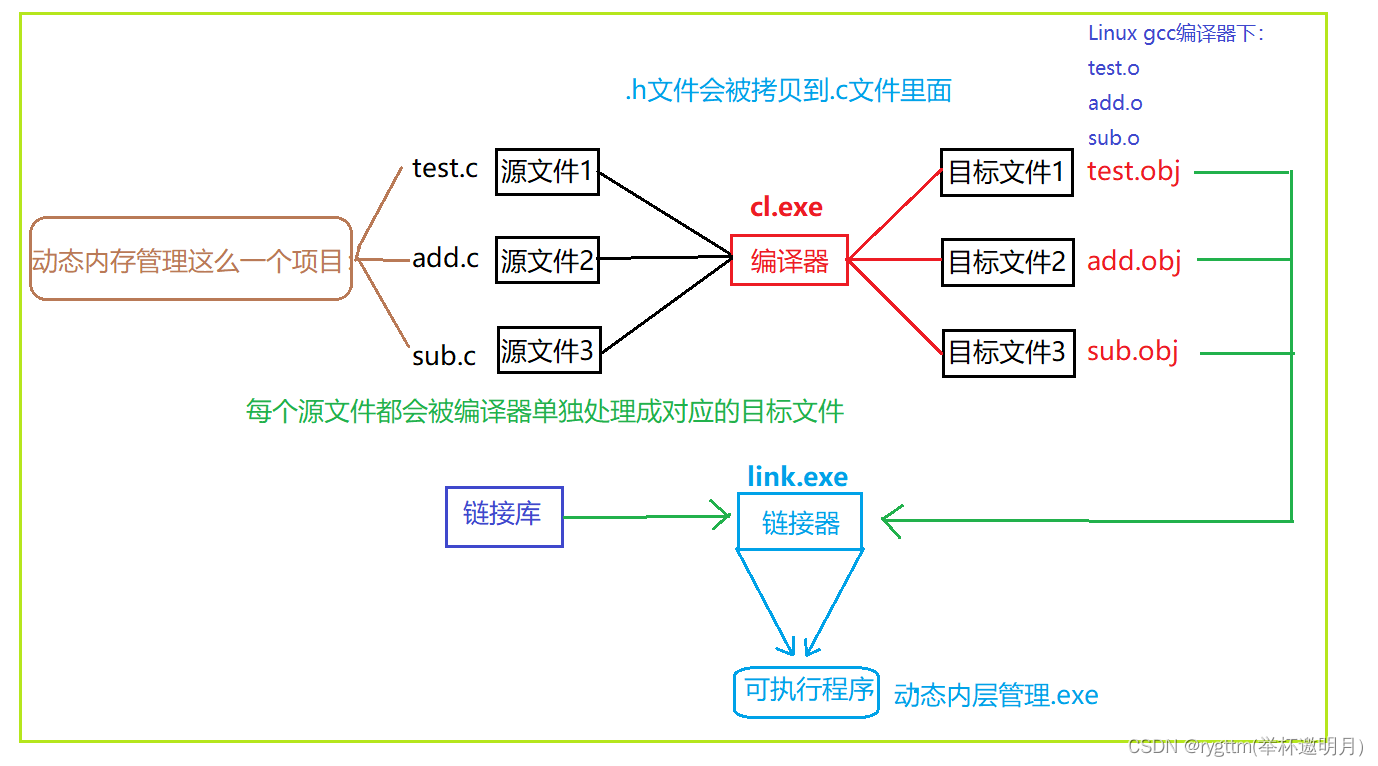a.在一个工程当中，由于需求的多种多样，程序猿往往要写出很多很多的代码，并且不同的程序猿所在的小组需要完成的任务也不一样，那么在这个工程当中就一定会出现很多的源文件，等到所有小组完成任务之后产生这么一个.exe的可执行程序文件。

b.但从代码到可执行程序的过程中要经过的工作可是太多了，总不能从代码直接变出来一个可执行程序吧，我们下面就详细的介绍翻译环境中，程序从代码开始，要经历什么养的步骤。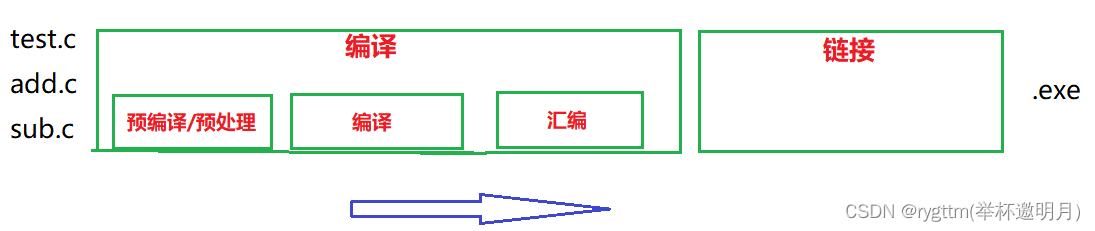1.预编译：gcc test.c -E -o test.i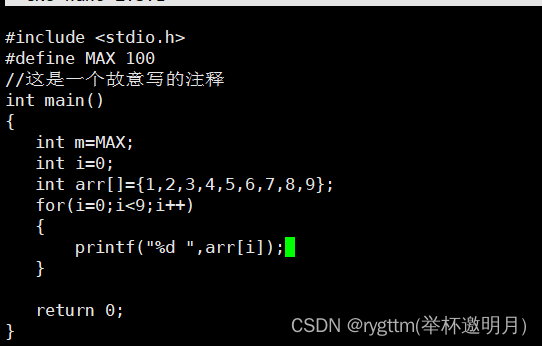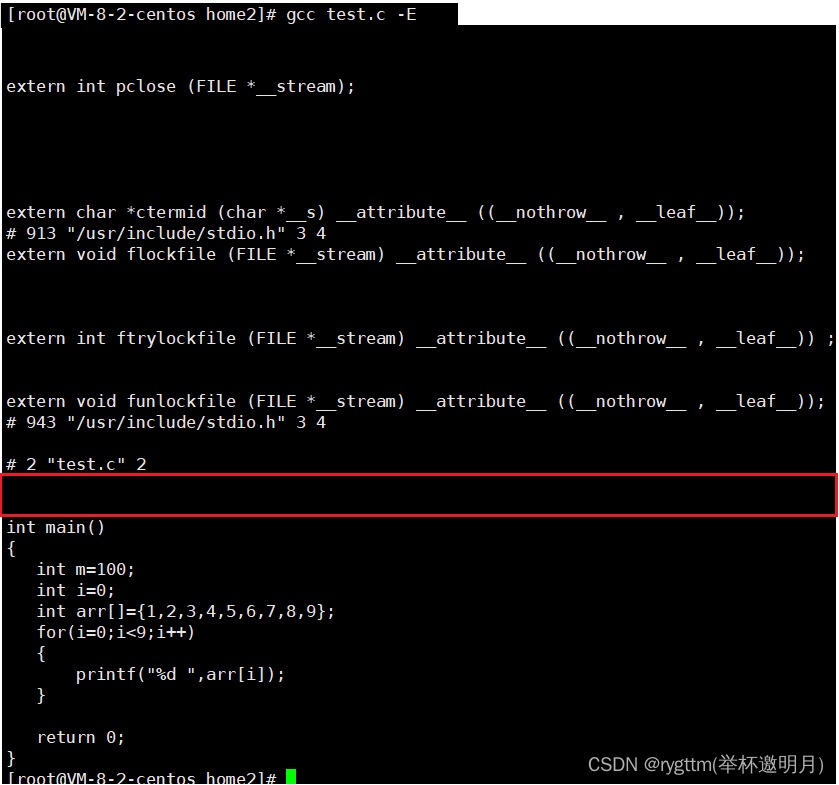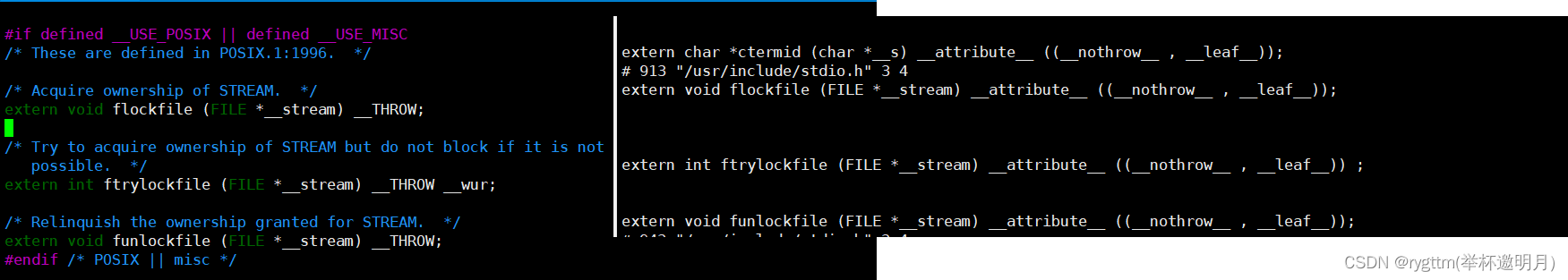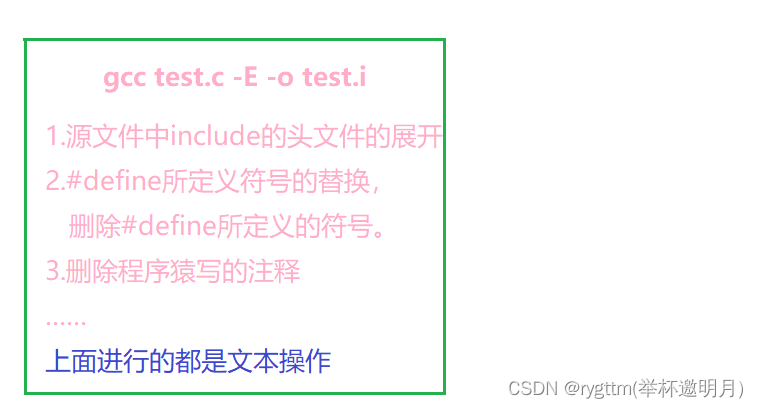2.编译：gcc test.i -S

gcc编译代码过后会产生一个test.s的文件，test.s文件中的内容其实就是预编译、编译阶段过后产生的文件。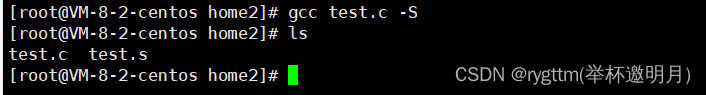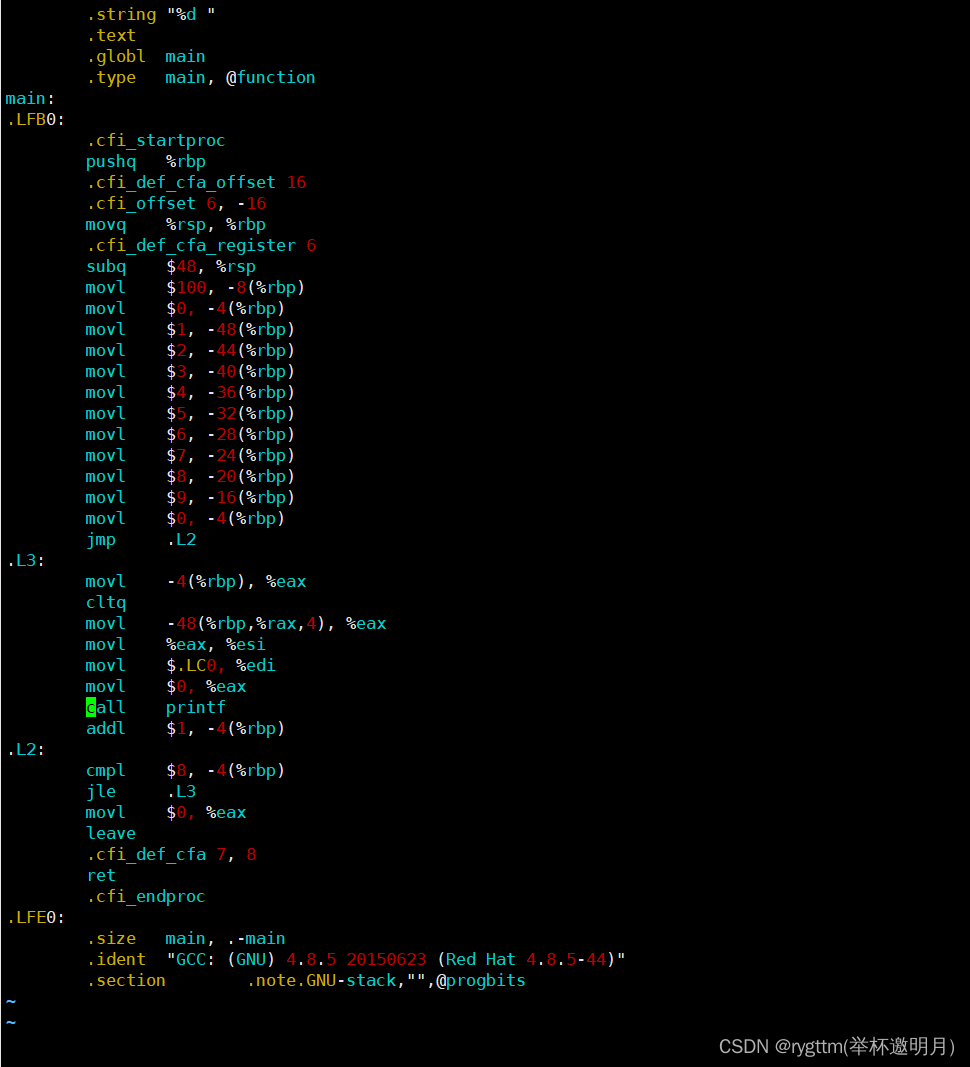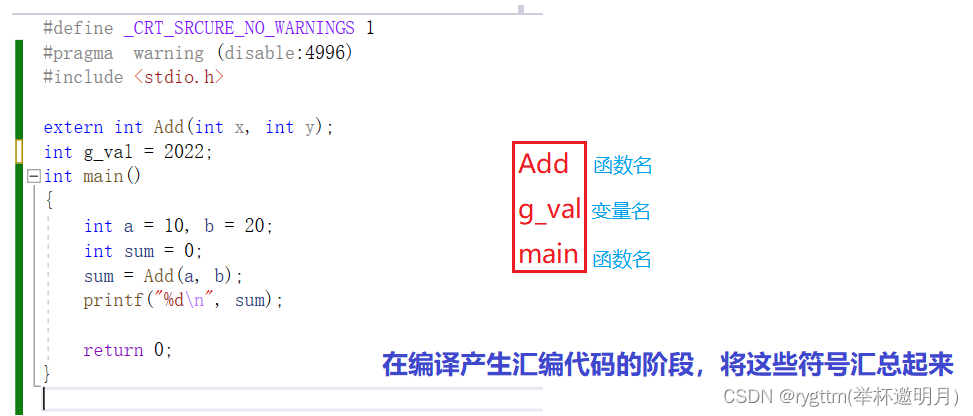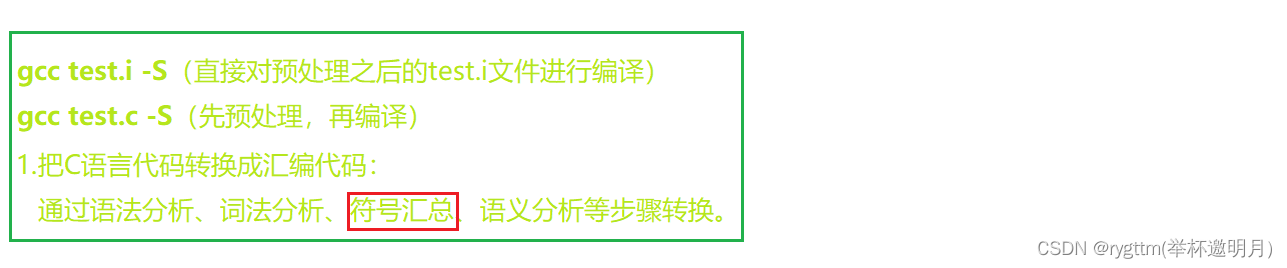3.汇编：gcc test.s -c（生成可重定位目标二进制文件）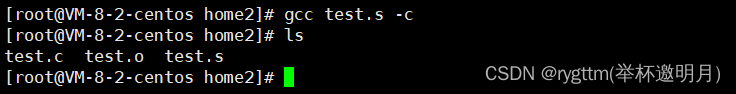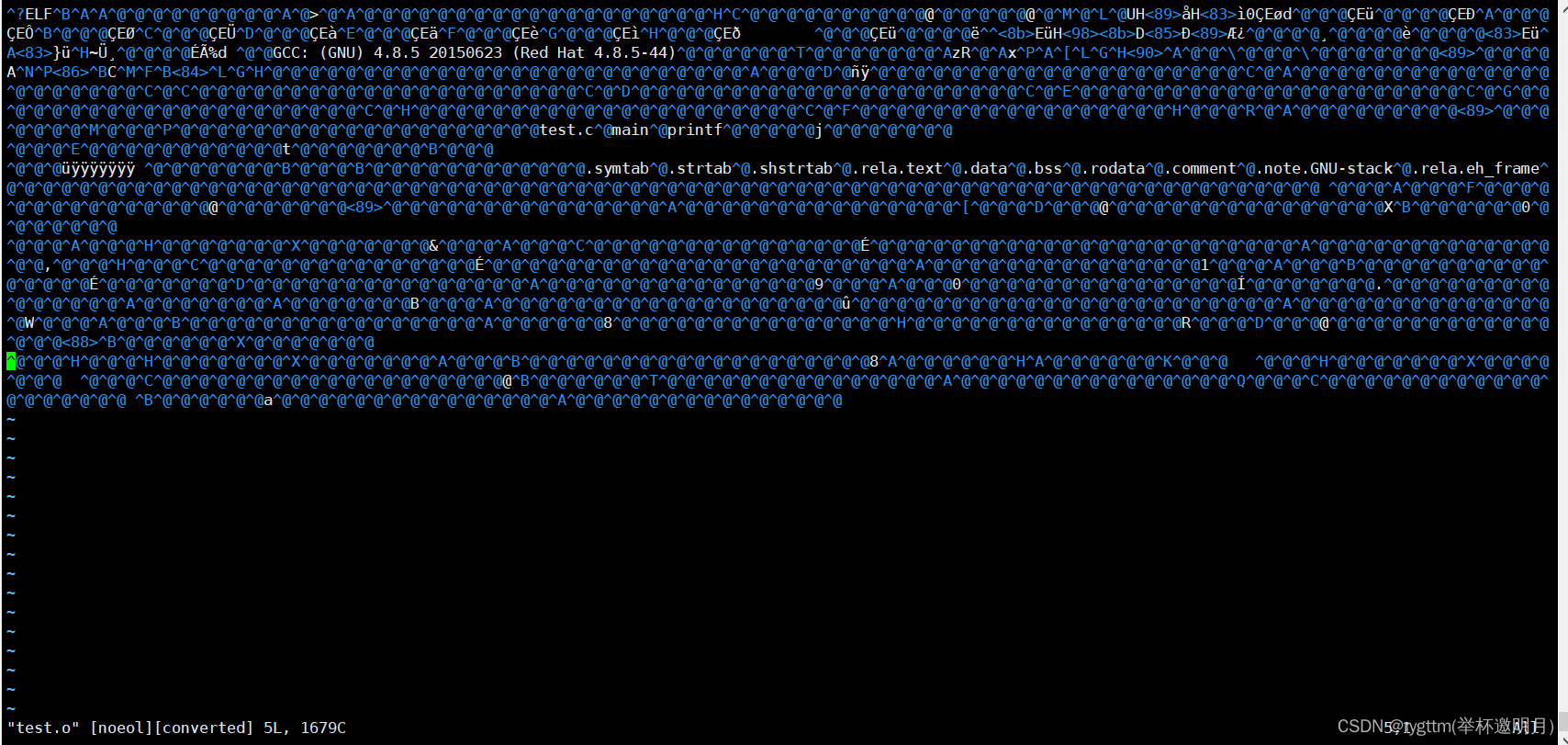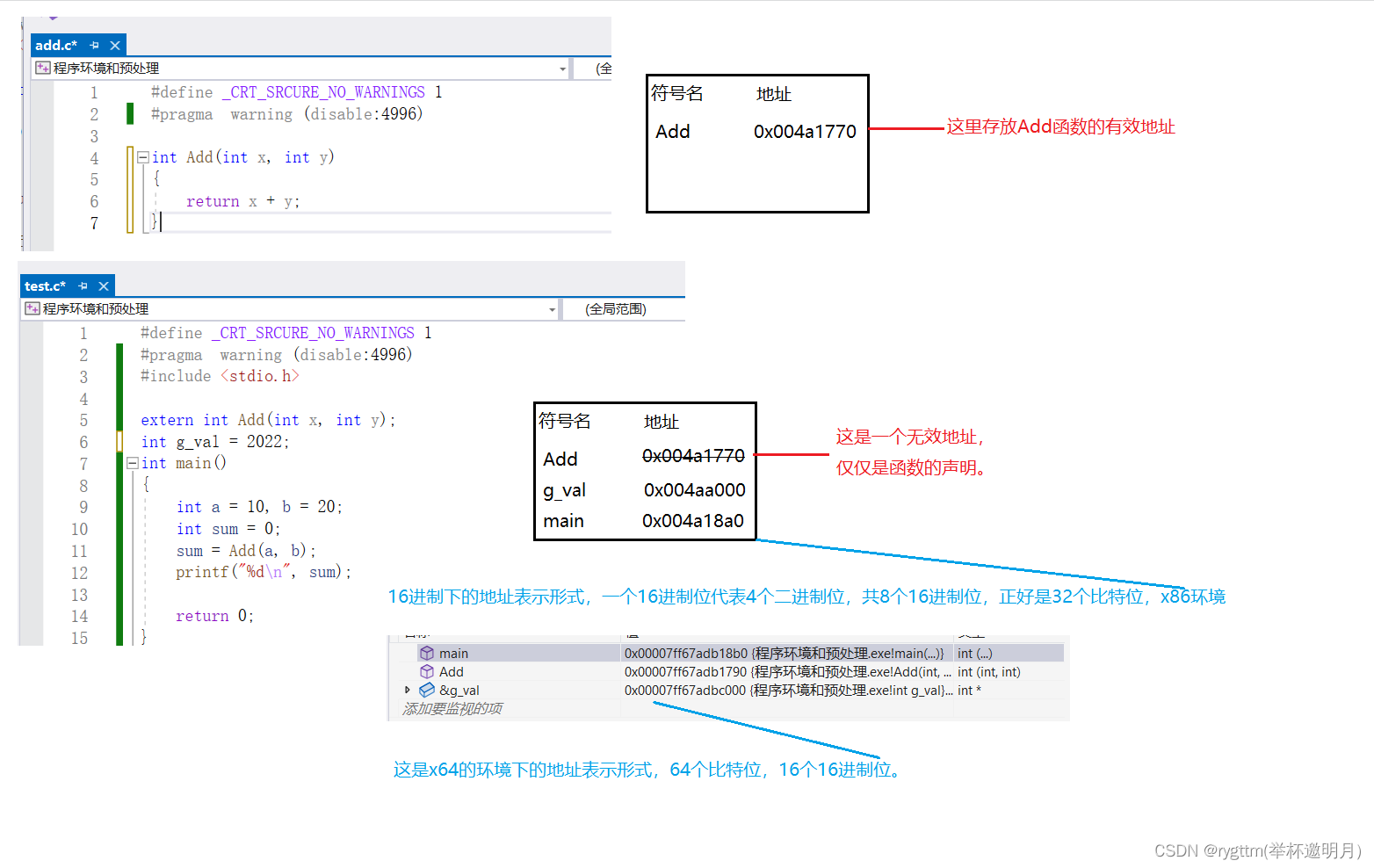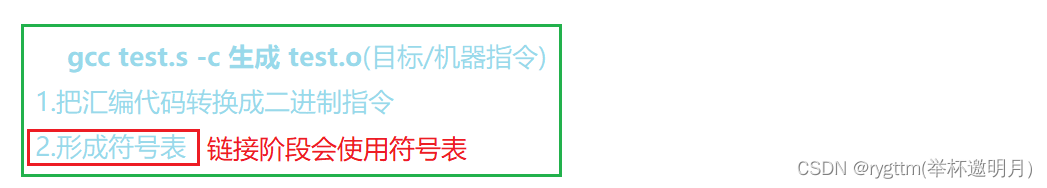4.链接：符号表的合并（很重要）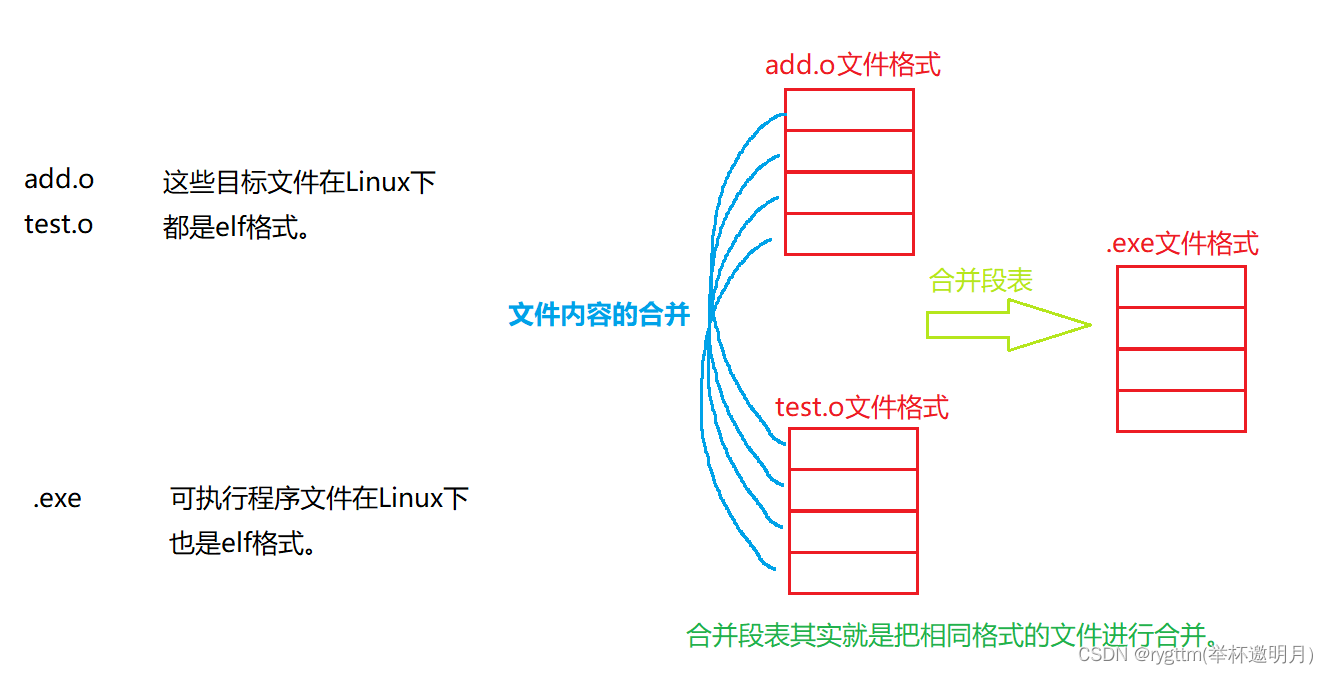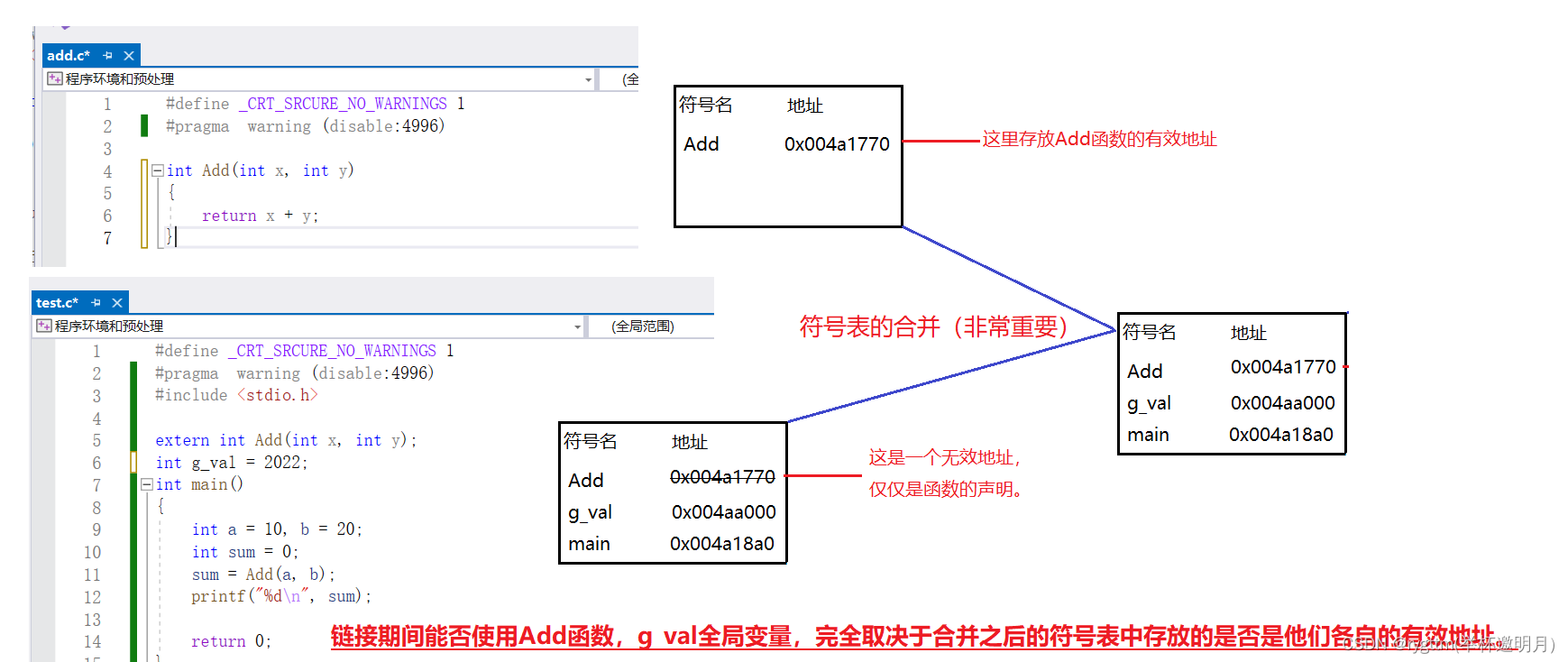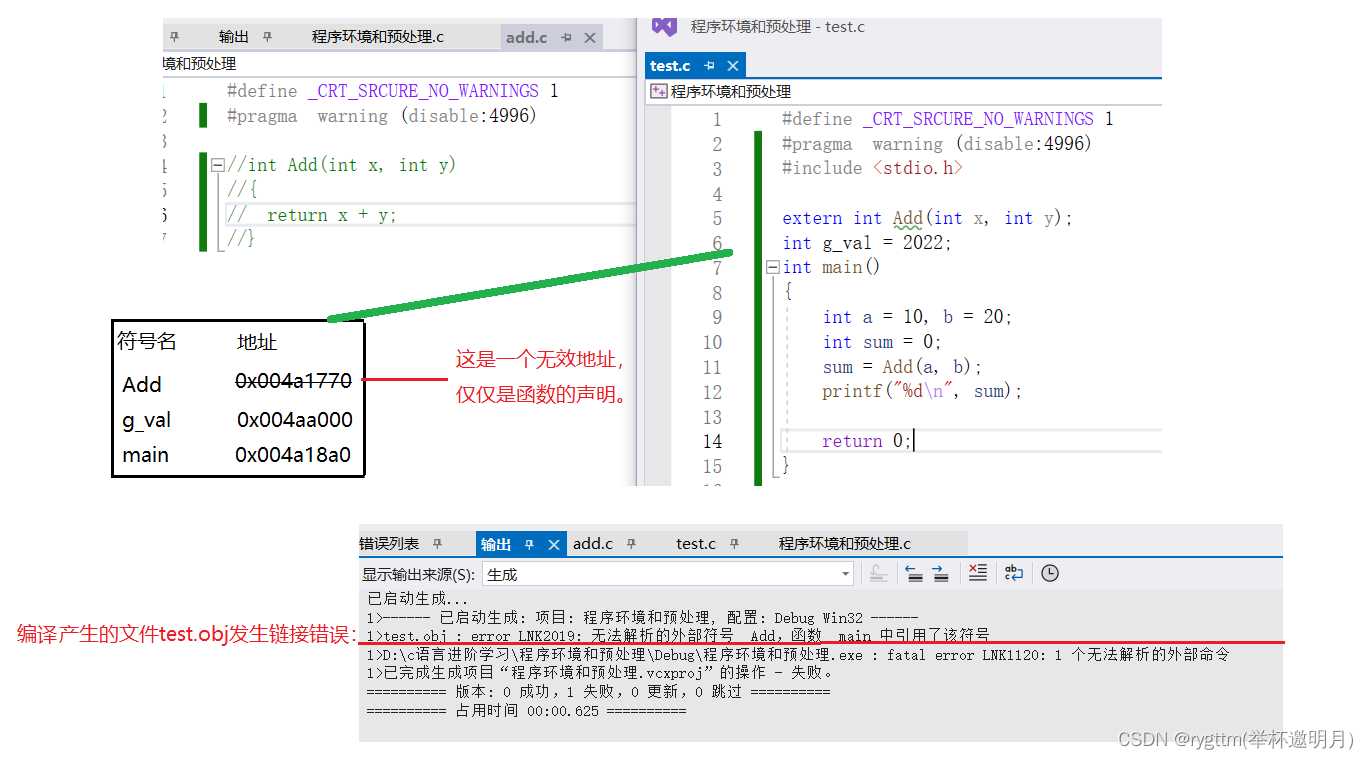1.程序必须载入内存中。

2.开始执行程序：

3.开始执行程序代码：

4.终止程序：

# 二、预处理（预编译）阶段展开讲解

## 1.C语言内置预定义符号

``````__FILE__    进行编译的源文件
__LINE__    文件当前的行号
__DATE__    文件被编译的日期
__TIME__    文件被编译的时间
__STDC__    如果编译器遵循ANSI C，其值为1，否则未定义

int main()
{
int i = 0;
for (i = 0; i < 10; i++)
{
printf("File:%s line=%d date:%s time:%s i=%d\n", __FILE__, __LINE__, __DATE__, __TIME__, i);
}
return 0;
}
``````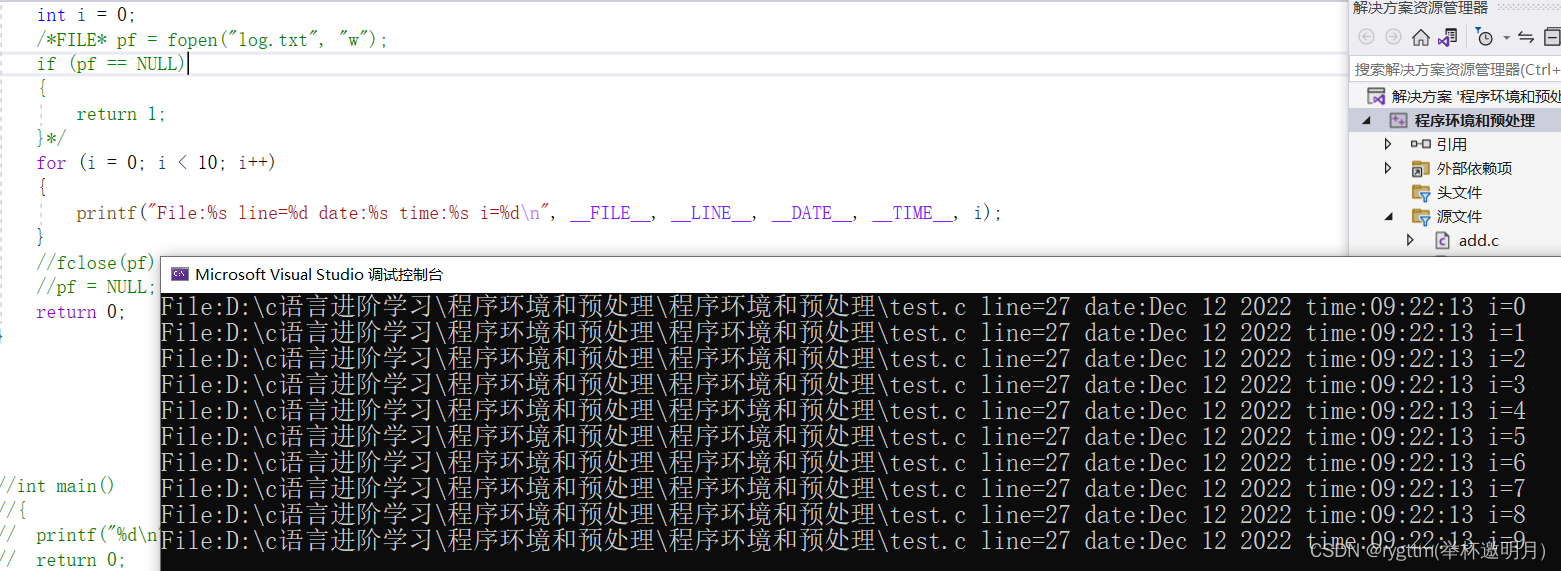``````int main()
{
int i = 0;
FILE* pf = fopen("log.txt", "w");
if (pf == NULL)
{
return 1;
}
for (i = 0; i < 10; i++)
{
printf("File:%s line=%d date:%s time:%s i=%d\n", __FILE__, __LINE__, __DATE__, __TIME__, i);
}
fclose(pf);
pf = NULL;
return 0;
}
``````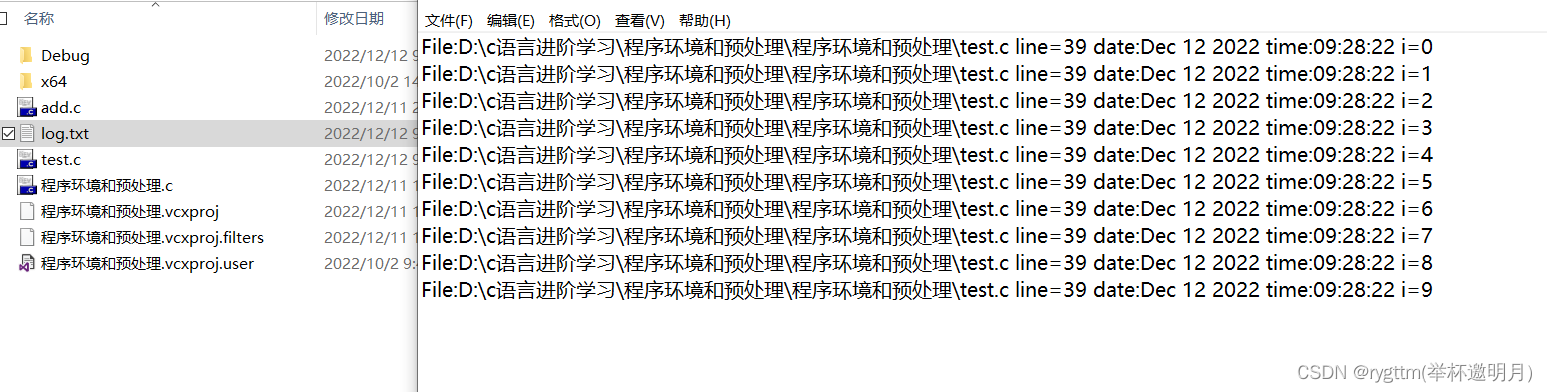vs不支持ANSI C 标准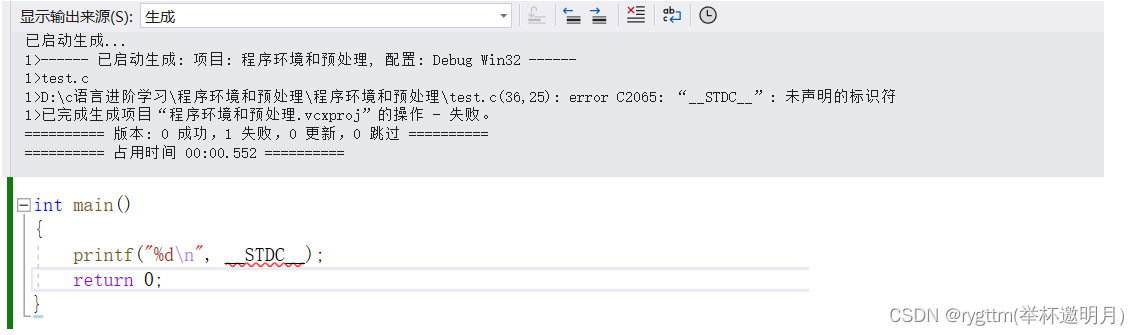gcc编译器是遵循ANSI C标准的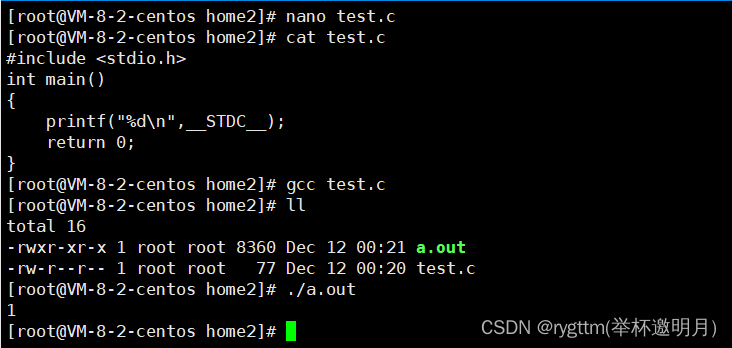## 2.#define定义标识符

``````#define MAX 1000
#define STR "hello wyn"
#define print printf("hello wyn\n")
//不要加分号，因为他是纯粹的替换

#define DEBUG_PRINT printf("file:%s\tline:%d\tdate:%s\ttime:%s\n",\
__FILE__,\
__LINE__,\
__DATE__,\
__TIME__)

int main()
{
printf("%s\n", STR);
printf("%d\n", MAX);
print;
DEBUG_PRINT;
return 0;
}
``````

1.在用#define定义标识符的时候，我们不要加；分号，这很容易导致下面使用标识符时，发生语法错误。
2.如果定义的名字过长，可以利用反斜杠\来当作续行符
3.额外的一个小知识点，vs上可以利用工程中的属性–>预处理器–>预处理到文件–>test.i文件，我们便可以在文件中查找到预处理之后的代码

## 3.#define定义宏（带有参数）

#define除可以用来定义表示符外，还可以定义宏，与标识符不同的是，在定义宏时，#define机制允许我们将参数替换到文本中，这样的实现我们称之为宏。

``````#define SQUARE(X) X*X
#define SQUARE(X) (X)*(X)//修改之后的定义宏

int main()
{
int r = SQUARE(5);
printf("%d\n", r);

r = SQUARE(5+1);//你以为答案是6，可惜答案是11
// 5 + 1 * 5 + 1
printf("%d\n", r);

return 0;
}

#define DOUBLE(X) (X)+(X)
#define DOUBLE(X) ((X)+(X))//定义宏的时候，不要吝啬括号

int main()
{
int r = DOUBLE(3 * 2);
printf("%d\n", r);

r = 10 * DOUBLE(3);//你以为答案是60，可惜答案是33
//10*(3)+(3)
//用修改之后的宏，替换后就是10*((3)+(3))，就是你想要的答案60
printf("%d\n", r);

return 0;
}
``````

## 4.#define所定义的标识符和宏的替换规则

a. 在调用宏时，首先对宏参数进行检查，看看是否包含由#define定义的标识符。如果有，标识符首先会被替换掉。

``````#define M 100
#define DOUBLE(X) ((X)+(X))
int main()
{
"M";//这些常量字符串如果和宏或标识符重名，预编译阶段是不会被替换的。
"DOUBLE(3)";//都不会被替换
DOUBLE(M + 2);
//替换
//((100+2)+(100+2))
return 0;
}
``````

b. 代码中使用宏的地方，会被我们定义宏时的替换文本给替换掉。

c. 最后，再次对结果文件进行扫描，看看它是否包含任何由#define定义的符号。如果是，就重复上述过程。

## 5.#和##的作用（替换为字符串 && 合并两边宏参数）

1.#的作用：并不是宏参数的单纯替换，而是替换为带有宏参数的字符串

``````//我们先来看这样一段代码，如果你运行后，会发现结果其实是一模一样的，
//所以字符串其实是具有自动链接的特点的
int main()
{
printf("hello wyn\n");
printf("hello ""wyn\n");

return 0;
}
``````

``````void print(int n)
{
printf("the value of n is %d\n", n);//传过来的是一个参数怎么完成下面那样的功能呢？
//这时候就要用到我们所学的宏了。
}
int main()
{
int a = 10;
printf("the value of a is %d\n", a);
int b = 20;
printf("the value of a is %d\n", b);
return 0;
}
``````

``````#define PRINT(N) printf("the value of " #N " is %d\n",N)
//#N会被预处理为"N"也就是被处理为字符串，然后替换。
int main()
{
int a = 10;
PRINT(a);
int b = 20;
PRINT(b);
return 0;
}
``````

2.##的作用：合并左右两边的宏参数

``````#define CAT(word,name) word##name
int main()
{
int lovewyn= 100;
printf("%d\n", CAT(love, wyn));
return 0;
}
``````

## 6.带副作用的宏参数（a+1 &&a++）

``````#define MAX(a,b) ((a)>(b)?(a):(b))

int main()
{
int a = 5, b = 4;
int m = MAX(a++, b++);

printf("m=%d\n", m);
printf("a=%d b=%d\n", a, b);

答案：m=6,a=7,b=5
return 0;
}
``````

## 7.宏和函数对比+两者命名的约定

a.宏比函数在程序运行速度和性能开销方面更好一些。

#define MAX(a, b) ((a)>(b)?(a):(b))

b.宏是与类型无关的，它只负责替换

c.宏的参数中可以直接出现类型

``````#define MALLOC(num,type) (type*)malloc(sizeof(type)*(num))
int main()
{
MALLOC(10, int);//如果想用malloc开辟空间，我们可以直接使用宏
return 0;
}
``````

a.宏较长的话，可能大幅增加程序的长度

b.宏是无法调试的

c.宏由于类型无关，也就导致它不够严谨

d.宏可能会带来运算符优先级的问题，容易导致程序出现错误

e.宏的参数可能带有副作用&&宏不可以递归

offsetof – 宏 getchar – 宏

## 8.#undef（有点儿鸡肋）

``````#define M 100
int main()
{
printf("%d\n", M);
#undef M
printf("%d\n", M);
return 0;
}
``````

# 三、命令行定义（不在代码中定义符号）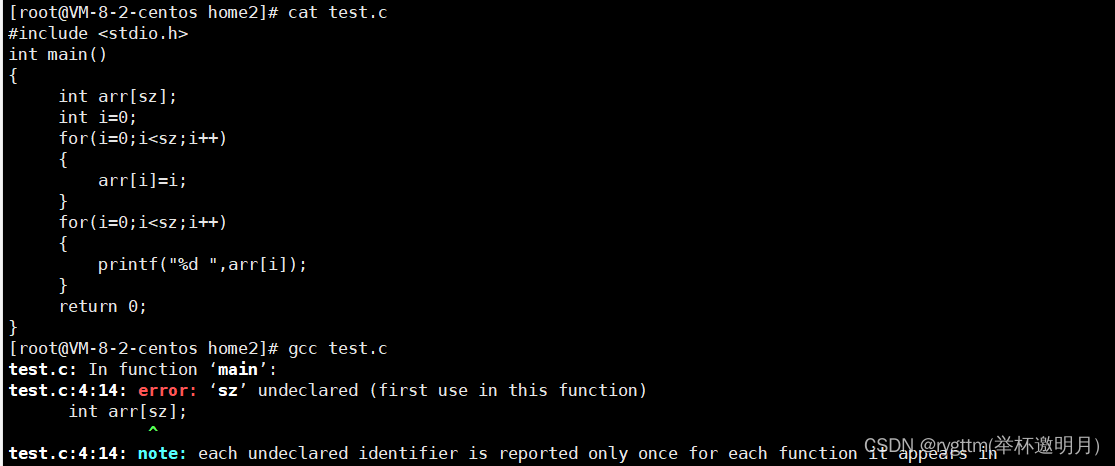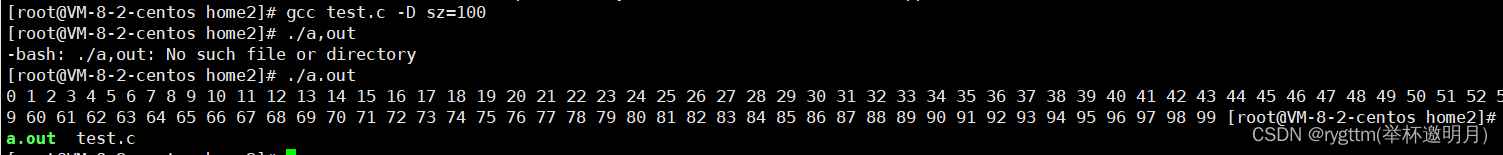# 四、条件编译（随心所欲的编译代码）

``````#include <stdio.h>
#define __DEBUG__
int main()
{
int i = 0;
int arr;
for (i = 0; i < 10; i++)
{
arr[i] = i;
#ifdef __DEBUG__ //这个地方判断为真endif和ifdef之间的语句才会参与编译，否则不参与编译
printf("%d ", arr[i]);
#endif
}
return 0;
}
``````

a.单分支条件编译

#if 常量表达式（不可以含有变量哦）
//…
#endif
//常量表达式由预处理器求值。

``````int main()
{
#if 2==3 //逻辑表达式，判断为true，参与编译，判断为false，不参与编译。
printf("hehe");
#endif
}
``````

b. 多分支条件编译

#if 常量表达式
//…
#elif 常量表达式
//…
#else
//…
#endif

``````#define M 3
int main()
{
#if M<5
printf("hehe\n");//预处理阶段这些预定义的东西都会被删除
#elif M==5
printf("haha\n");
#else M<5
printf("heihei\n");
#endif
return 0;
}
``````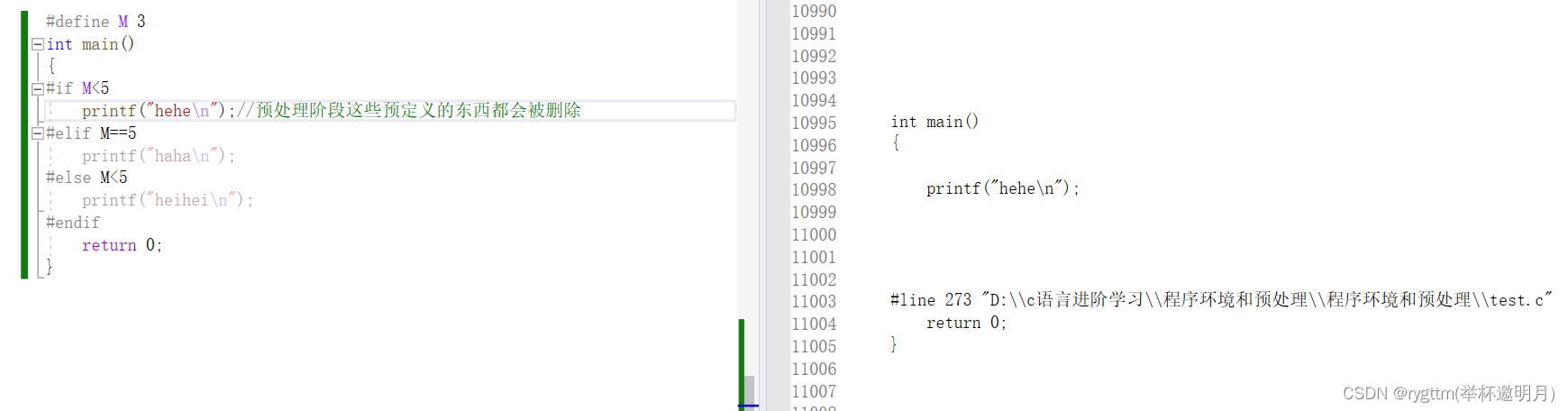c.判断是否被定义

``````#define MAX 1000
int main()
{
#if defined MAX
printf("max\n");
#endif

#if !defined MAX//!反逻辑操作符，入伏哦没定义MAX，我们输出max语句
printf("max\n");
#endif
//上下两段代码表达的意思都是相同的，只是写法上有些不同而已，随便选一种写法即可。
#ifdef MAX
printf("max\n");
#endif

#ifndef MAX//反逻辑的另一种写法#ifndef
printf("max\n");
#endif
return 0;
}
``````

d.嵌套指令

``````#if defined(OS_UNIX)

#ifdef OPTION1
unix_version_option1();
#endif

#ifdef OPTION2
unix_version_option2();
#endif

#elif defined(OS_MSDOS)

#ifdef OPTION2
msdos_version_option2();
#endif

#endif
``````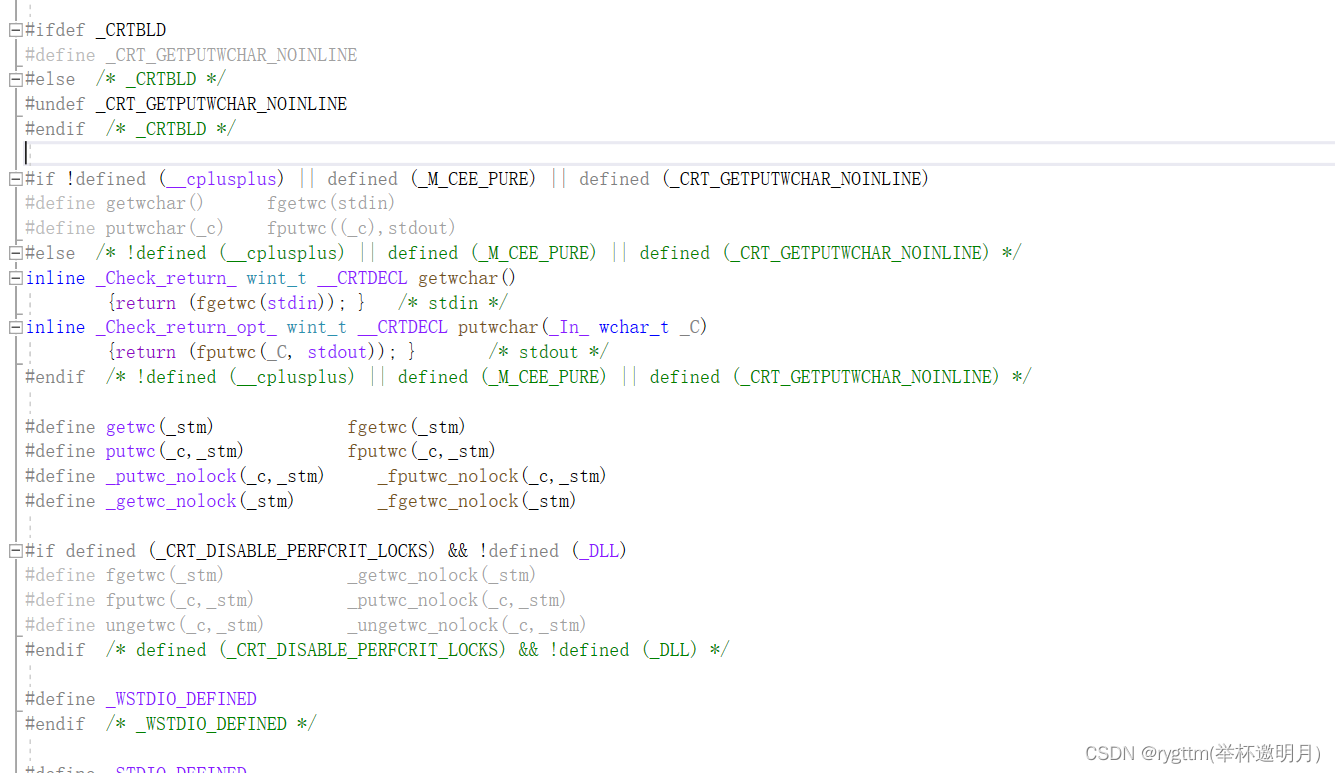# 五、文件包含（< >包含 && " "包含）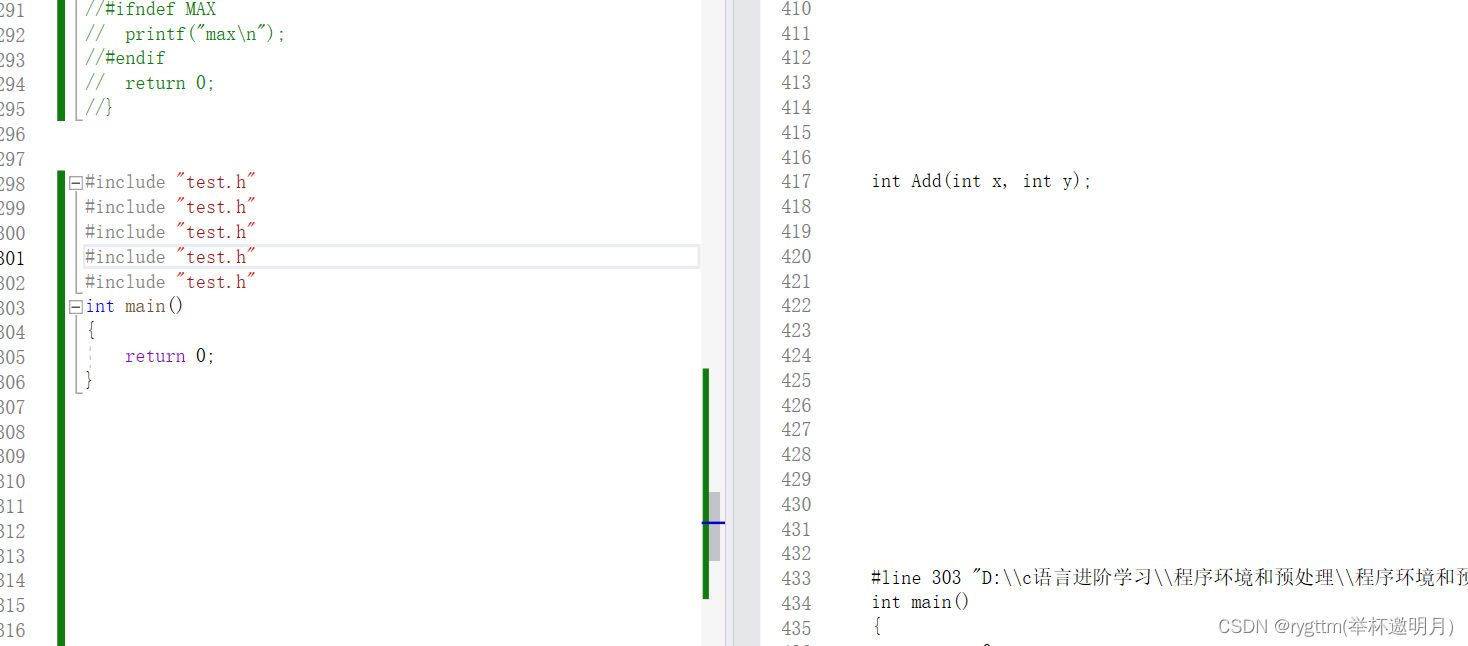a.利用条件编译指令

``````//防止头文件被重复多次包含
#ifndef __TEST_H
#define __TEST_H
#endif
``````

b.利用预处理指令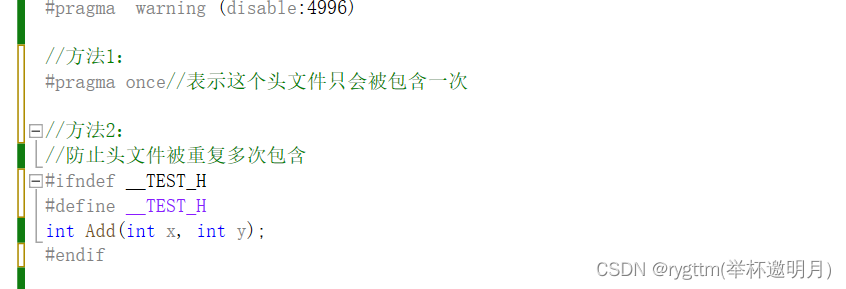<>和"“两种文件包含方式，其实就是在查找的方式上不同。
1.<>直接去include的库目录下去查找我们的头文件
2.”"先去代码所在的路径下面查找，如果找不到在去库目录下查找

# 六、offsetof宏的实现

``````#include <stdio.h>
#include <stddef.h>

struct S
{
char c1;
int i;
char c2;
};
#define OFFSETOF(type,M_name) (size_t)&((type*)0)->M_name //如果地址从0开始，那么地址强制类型转换后就是成员的偏移量
//默认对齐数是8，取较小的作为对齐数
int main()
{
struct S s = { 0 };
printf("%d\n", OFFSETOF(struct S, c1));
printf("%d\n", OFFSETOF(struct S, i));
printf("%d\n", OFFSETOF(struct S, c2));//总共占了9个字节，但得是4的倍数，所以结构体大小是12字节
printf("%d", sizeof(struct S));//答案就是12

//printf("%d\n", offsetof(struct S, c1));
//printf("%d\n", offsetof(struct S, i));
//printf("%d\n", offsetof(struct S, c2));//总共占了9个字节，但得是4的倍数，所以结构体大小是12字节
//printf("%d", sizeof(struct S));//答案就是12
return 0;
}
``````

# 七、交换整数二进制位的奇数位和偶数位

``````#include <stdio.h>

#define CHANGE(N) ((N&0xaaaaaaaa)>>1)+((N&0x55555555)<<1)
int main()
{
int num = 0;
scanf("%d", &num);
printf("%d", CHANGE(num));
}

``````

THE END